Sverige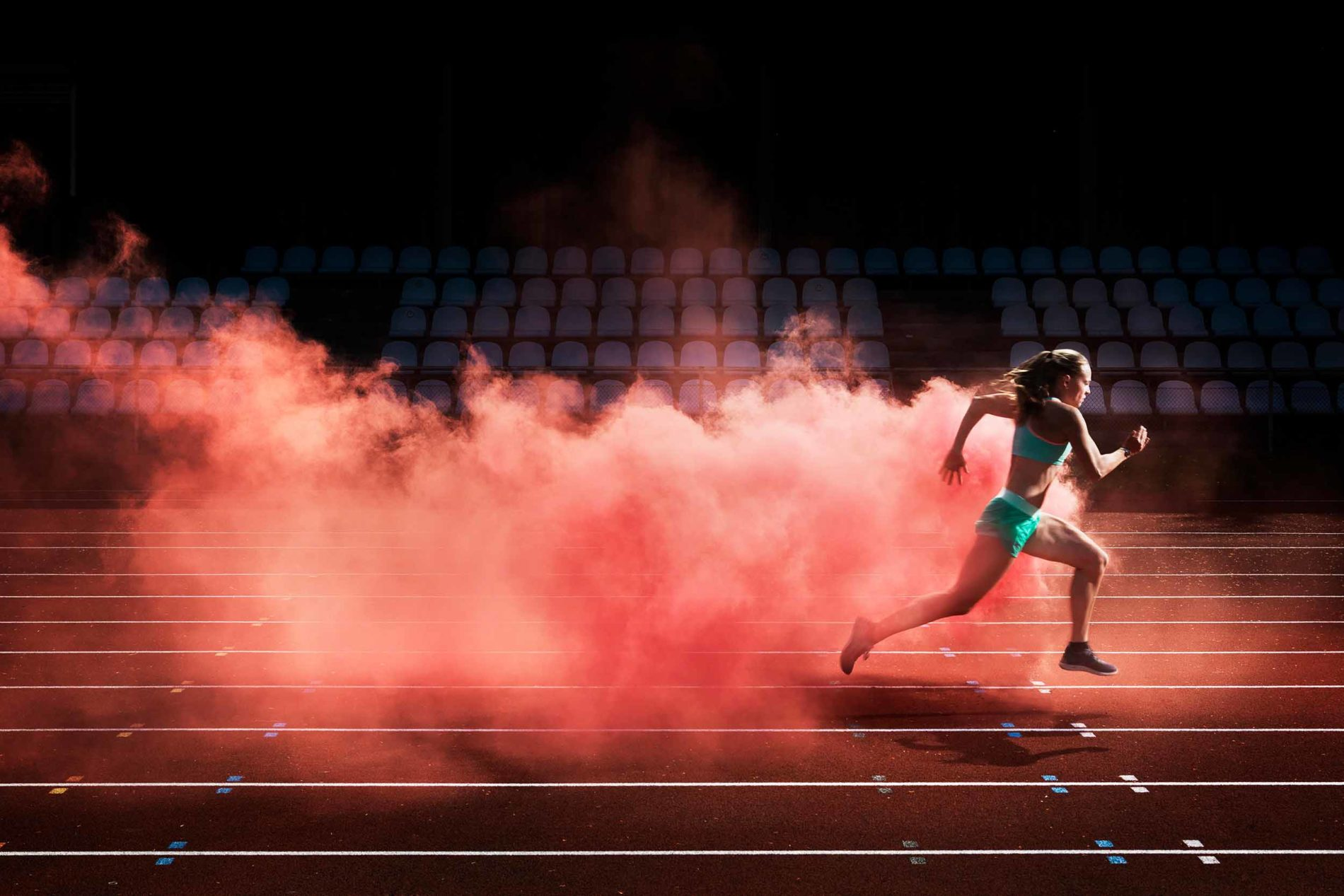# Do Olympic athletes become faster, stronger, and taller each year? We analyzed the data

As most of you are aware the postponed 2020 summer Olympic Games in Tokyo took place this summer. What many of you might not be aware is that during that same time, four prospective data analysts waited for their first day at Sopra Steria. Much like this summer’s Olympics, which took place with empty stands due to the Covid-19 pandemic, the first few weeks of work for these analysts were not very busy. Therefore, we decided to spend our time doing some analysis and visualizations on a dataset with information of historical data from the Olympics to see if we could see any trends or could draw any interesting conclusions.

## The Data

The dataset consists of information on athletes that have competed in the Olympics between 1896 and 2016 and contains is 271 116 entries.

There are 15 datapoints for each athlete and year, however we were not interested in all of those for our analysis. The fields we were interested in were those with information about the height and weight of the athlete, which year they competed, which event they competed in and whether they received a medal or not. This means our first step was filtering out all the entries which don’t have values in the height and weight columns which would leave us with 206 853 entries. However, before dropping the data, it is often a good idea to visualize which data will be dropped, as this might impact later analysis. Here we looked at how many values are missing per year and the average percentage of dropped entries per sport.

The goal was to see if there are any patterns in the missing values, and we could clearly see that there are some events that have an average of 100% missing height and weight values. We could also see that there were a lot of missing values from earlier dates. As a lot of our analysis will study the difference in years and events, we filtered out all data where an event had over 50% missing values for that year. More advanced analysis and imputation for the missing values is possible but is beyond this blogpost. After filtering the data, we were left with 199196 entries.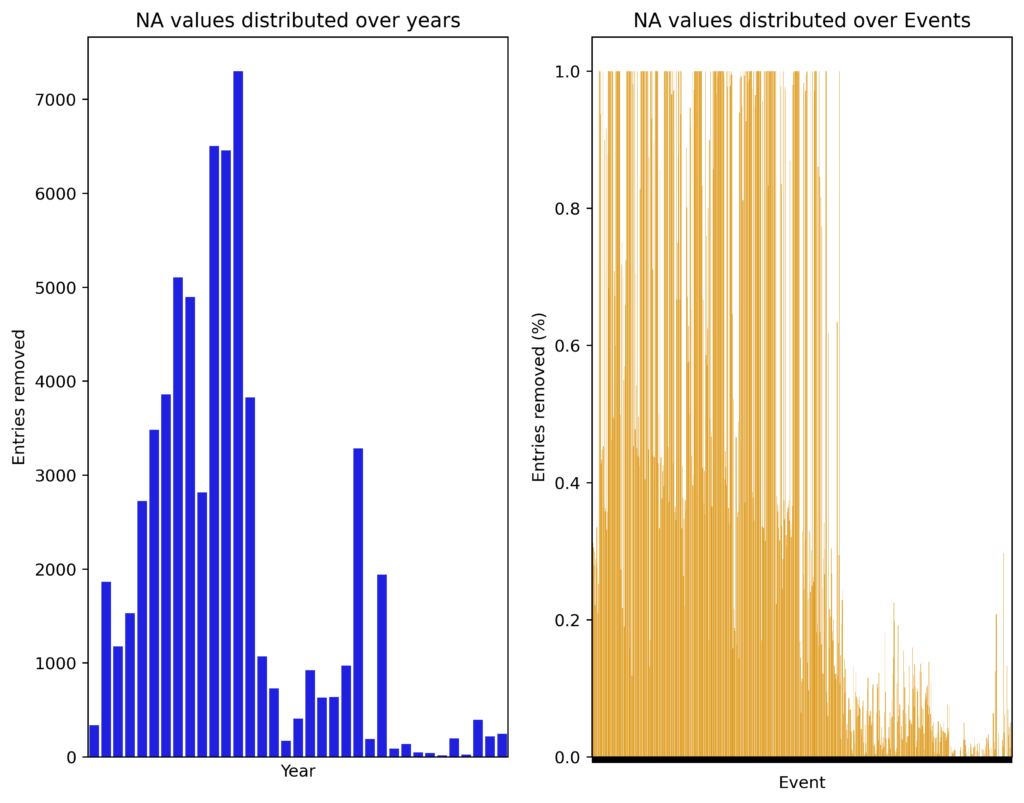## Visualizing the data

After the initial cleaning it was time to look at the data! Below is a graph showing the full dataset split by Gender and colored by which sport the athlete competed in.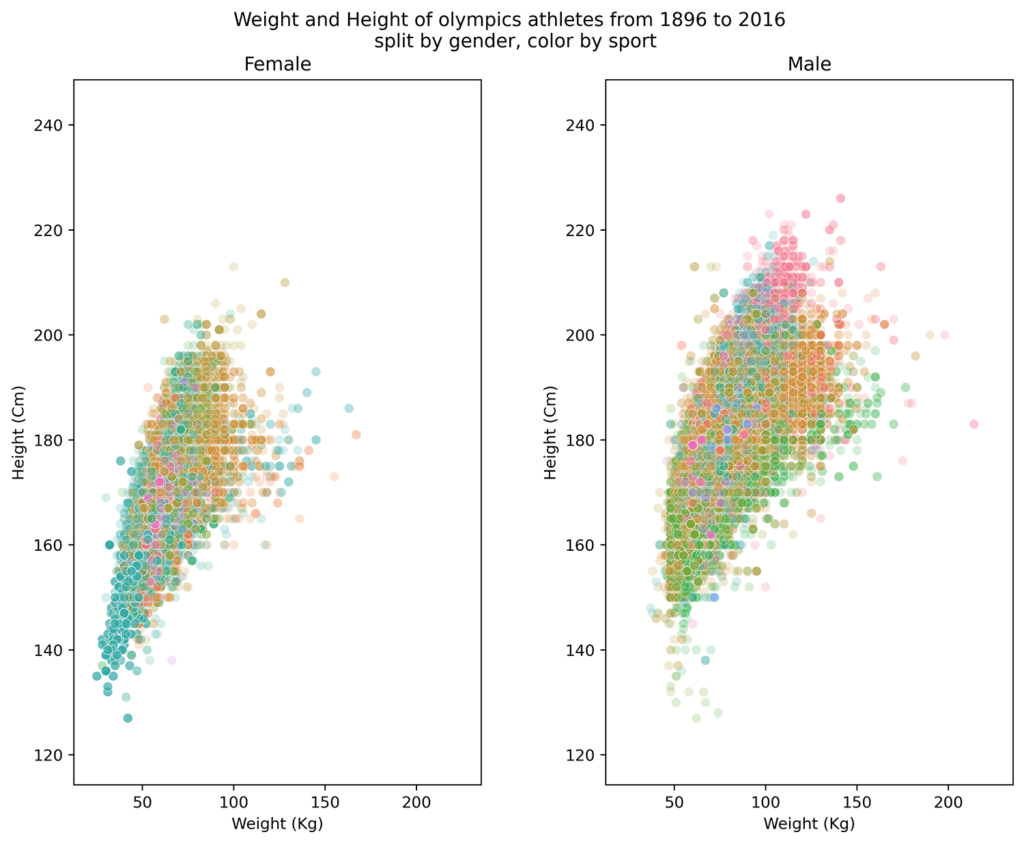These charts showed us that that there is some variety in both height and weight of the athletes. The colors also indicate that there might be some differences within sports, this however is quite difficult to say for sure without further analysis. One aspect that is not shown in these charts is how the values have evolved throughout the years. This can instead be seen in the Gifs we created shown below.

From these Gifs, we saw that since the beginning of the Olympics the athletes have become not only bigger but a more heterogeneous group in terms of size and weight.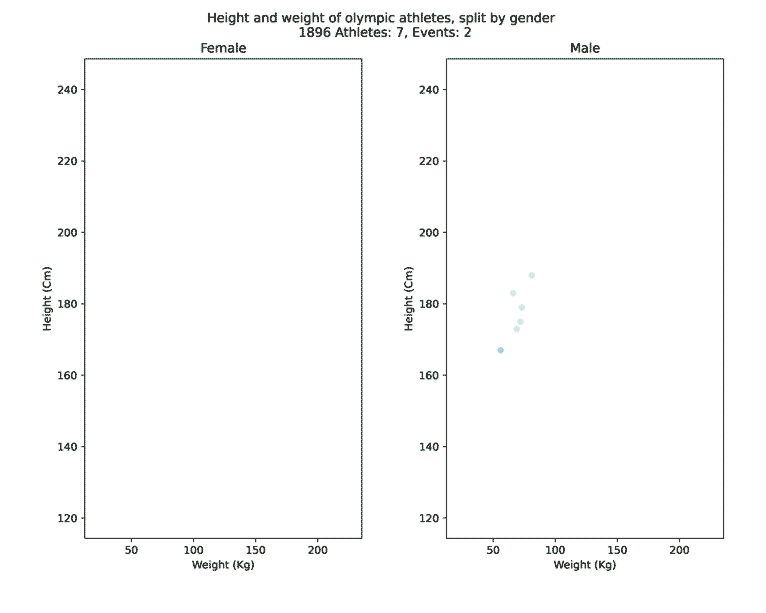## Comparing athletes from different sports

As we saw in our first plot there seems to be some difference between the athletes of different events, but how big is it? First, we needed to establish how we measure the difference, as there were many athletes in each event, but also two variables to account for. What we were looking for is something called a distance measure, the most straight forward way is to use what is called Euclidean distance between the means of the two variables for each group. More precisely we defined the distance between group a and b to be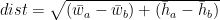$dist = \sqrt{ (\bar{w}_a - \bar{w}_b) + (\bar{h}_a - \bar{h}_b)}$  where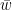$\bar{w}$  is the average weight, and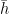$\bar{h}$ is the average height. Using this formula, we computed the distance between all the groups to find the ones with the biggest difference. For this we defined a group to consist of all the athletes that competed in an event for a certain year, for example would Men’s basketball, 2012, be a group. We also filtered out all groups with under 20 observations. We then visualized the results with figures, but for those of you who prefer numbers there is of course also a table.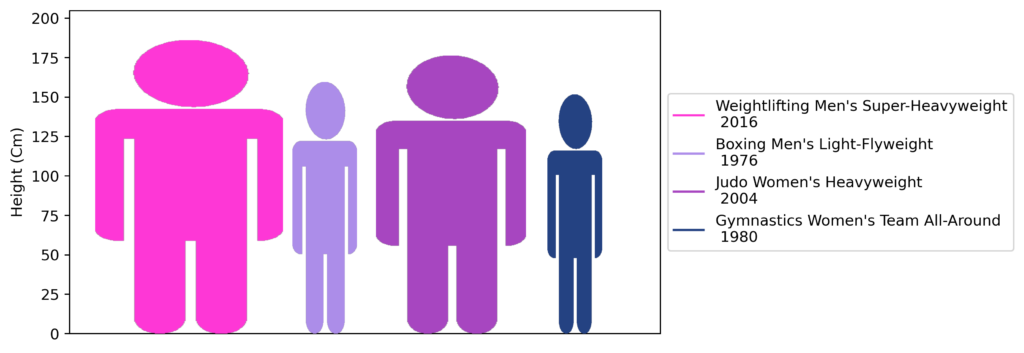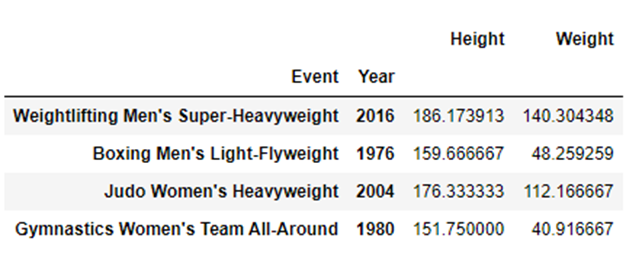## Calculating the trend in height and weight for each event

The next thing we wanted to analyze was how have athletes within sports changed over time. To do this we first needed to quantify a change over time for the athletes within a sport. We did this by running an ordinary linear regression of type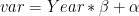$var = Year * \beta + \alpha$ where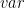$var$ is the variable we want to find the trend for, for example height or weight.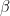$\beta$ can then be seen as the change in average for the athletes in the variable as the year increase. An example looks like this, in the below graph you can see that the trend for the height of athletes playing men’s basketball is an increase by 0.23 cm per year. While this might not sound like a lot over the 50 years it accumulates to a bit over 10 cm.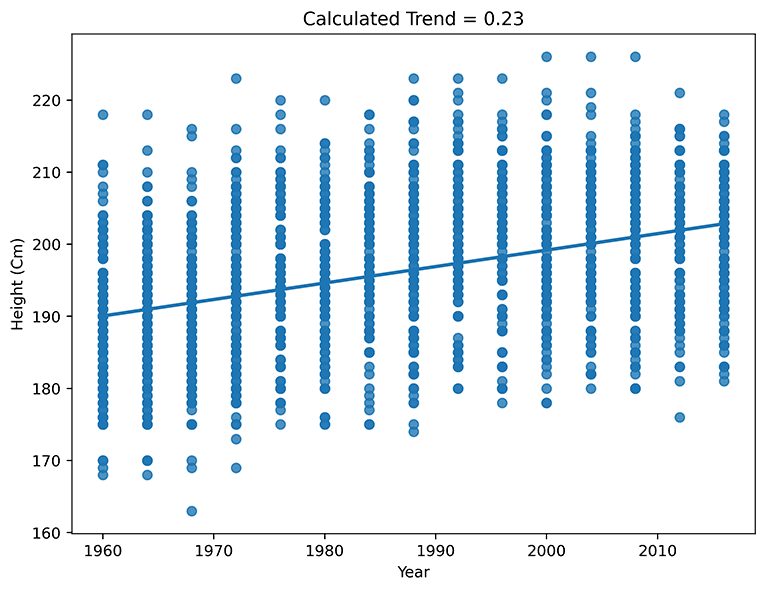Calculating the trend for both height and weight for each sport allowed us to visualize how athletes from all different Olympic events have changed over time.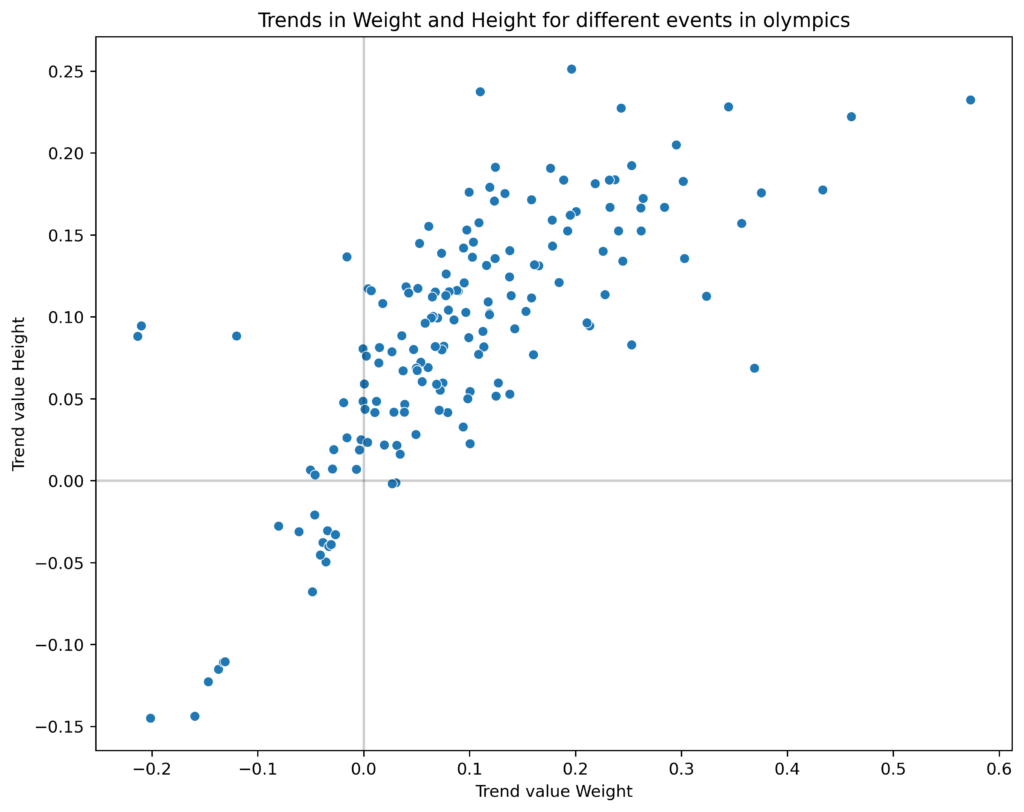Each dot in this plot represents an event and its placement in which direction the athletes have evolved in terms of height and weight. It becomes clear that for most of the events athletes have become taller and heavier and in almost no sports have they become shorter and heavier. There are also some sports where the athletes have become lighter and taller and some where they have become both shorter and lighter.

We then identified the events that was the furthest away from the center in each quadrant (excluding the fourth), i.e., the events that had changed the most over the years in each direction. This we did once again using the Euclidean distance. To visualize the evolution, we created two gifs showing the evolutions, one with labels and one without. For full transparency we should mention that we used the full data set when creating the gifs, while the analysis was done on the filtered data.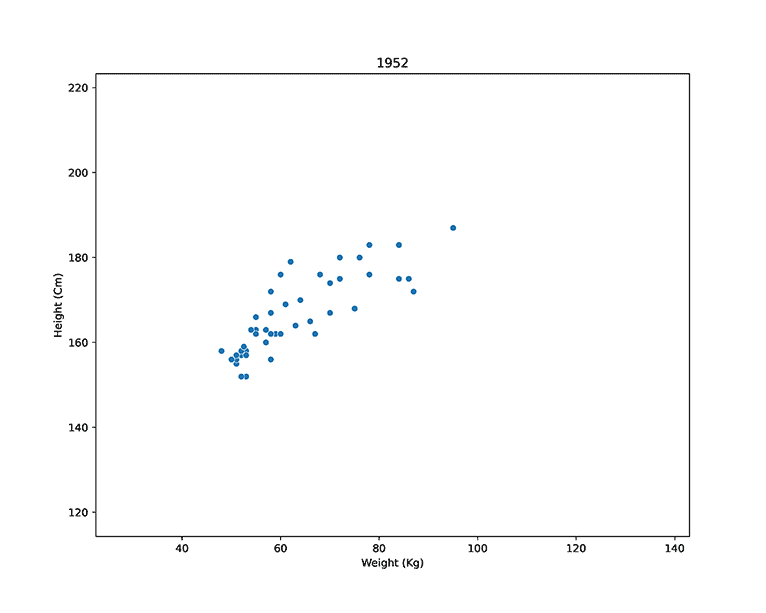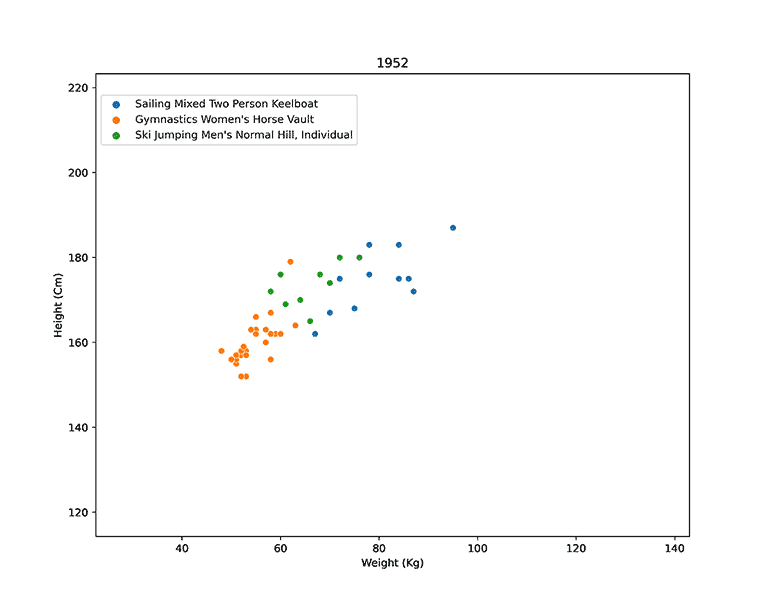## Analyzing performance of outliers

After the analysis on how height and weight have changed during the year, with clear indications that these values have changed over the years it would be pretty interesting to find out how height and weight correlates with how many medals athletes win. Is it an advantage to be of average height and weight or is it better to stand out amongst your peers?

In order to explore this, we first need to identify outliers based on height and weight. Because height and weight are measured in different scales and are likely to be highly positively correlated, Euclidean distance may not be optimal for this use case. Instead, we will use Mahalanobis distance which is defined as: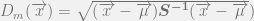$D_m(\overrightarrow{x}) = \sqrt{(\overrightarrow{x} - \overrightarrow{\mu})\boldsymbol{S^{-1}}(\overrightarrow{x} - \overrightarrow{\mu}})$  where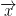$\overrightarrow{x}$  is a set av variables and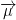$\overrightarrow{\mu}$is the mean of those variables, lastly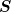$\boldsymbol{S}$ is the covariance matrix. Using the covariance matrix, Mahalanobis distance can manage correlated variables and different scales.

In order to obtain a fair comparison, the athletes are compared in groups where they are of the same sex and competed in the same event and year. If a particular group’s sample size is considered too small, they will not be evaluated.

The Mahalanobis distance from the mean is calculated for all observations. The Mahalanobis distance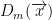$D_m(\overrightarrow{x})$ follow a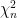$\chi^2_n$ distribution with$n$ degrees of freedom where$n$ is the number of variables, which is 2 in this case. From the observed mahalanobis distance and the$\chi^2_n$ distribution, p-values can be calculated. Observations with p-values less than 0,05 are considered outliers. The region where observations are classified as outliers can then be visualized by an ellipse.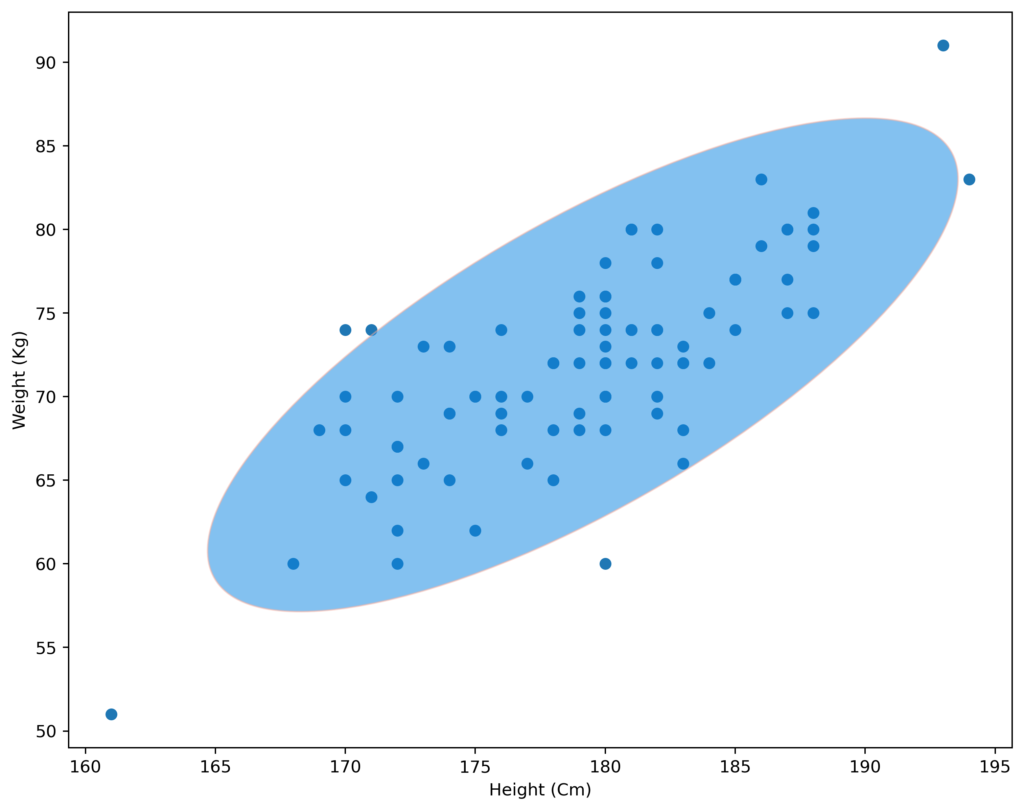Here we plotted height against weight for male athletes competing in the 2014 winter Olympics, in the event 20 kilometers Biathlon. From the plot we can identify 5 clear cut outliers and 2 that are just on the edge of the ellipse. We look at the 7 athletes with lowest p-values to examine.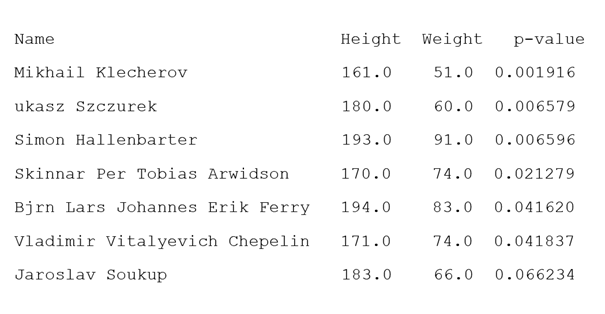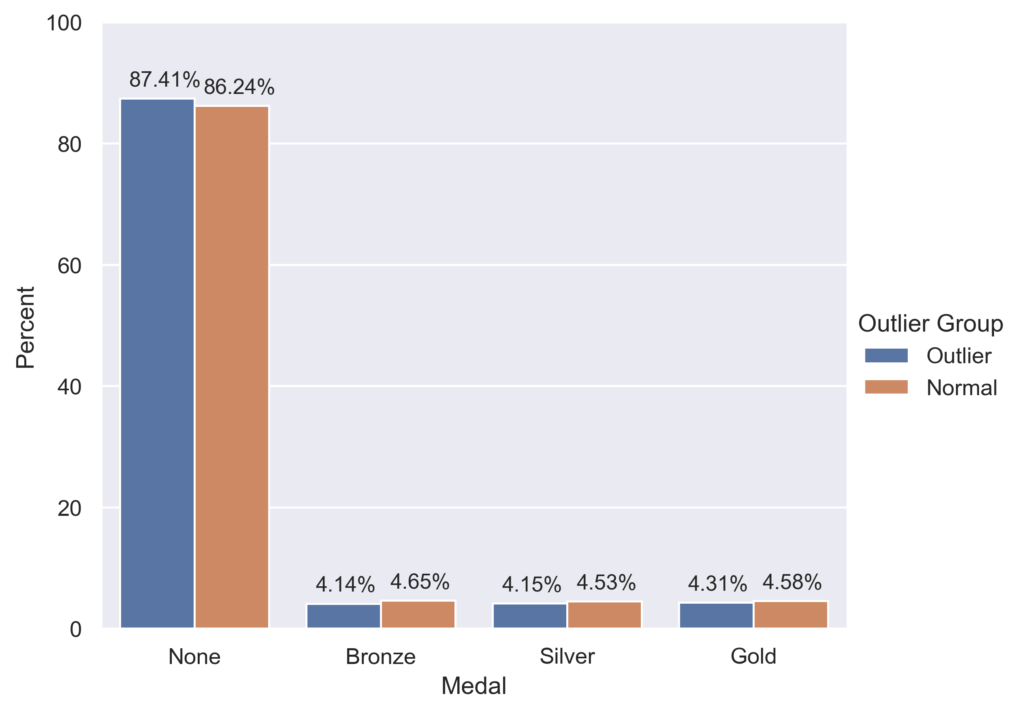Now we can clearly see that 6 athletes have p-values less than 0.05 and therefore are classified as outliers. For all the Swedes reading we can note that Björn Ferry, a Swedish legend in biathlon is classified as an outlier.

Now that we have classified athletes as either outliers or not, we can further analyze if outliers perform better or worse in the Olympics. We will look at the proportion of medals won for outliers and “normal” athletes to investigate this.

The bar plot visualizes the medals won, grouped on if the athlete is considered an outlier or not. From the bar plot we can conclude that outliers are less likely to win medals compared to non-outlier athletes. However, the differences are not very large, therefore we conduct a hypothesis test to see if there are significant evidence that the two groups are different.

The test used for determining this is a$\chi^2_n$ test of independence. This test is used to decide whether there exists a relationship between two variables in a population. The test uses a cross table which in this case looks like this.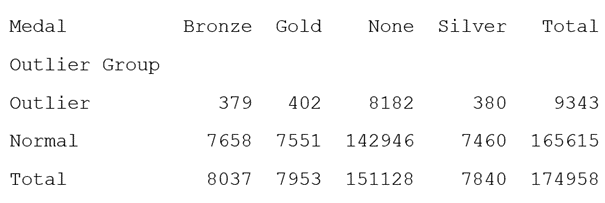The hypothesis’s used are:

•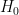$H_0$: Medal and outlier group are independent.
•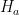$H_a$: Medal and outlier group are not independent.

Under the hypothesis that the variables Medal and outlier group are independent, the expected value for each cell is calculated from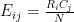$E_{ij} = \frac{R_iC_j}{N}$, where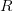$R$ and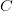$C$ stands for row and column total, and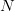$N$ stands for the grand total.

The test statistic is then calculated by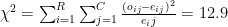$\chi^2 = \sum_{i=1}^R\sum_{j=1}^{C} \frac{(o_{ij} - e_{ij})^2}{e_ij} = 12.9$   , where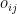$o_{ij}$ represents the observed values and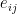$e_{ij}$ represents the expected values.

The test statistic is then compared to a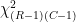$\chi^2_{(R-1)(C-1)}$ distribution.

With a significance level of 0.05 the critical value is found by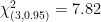$\chi^2_{(3,0.95)} = 7.82$

The test-statistic is greater than the critical value of the test. Therefore, we can conclude that there exists a statistically significant relationship between if you are an outlier or not and winning medals. In simpler terms, athletes who have similar weight and height as their peers are more likely to win medals at the Olympic games, compared to athletes in the same event and year.

## Summary

As we can see there is clearly a difference in athletes competing in different events, we can also see that there seems to be a trend where the height and weight of athletes from different events have evolved in different directions. Furthermore, we can see that being an outlier in terms of height and weight did not seem to improve chances for a medal. While it is certainly fun to analyze data on Olympic athletes it might seem far removed from something that creates values for businesses, which is what we work with. However, the exact same analysis that was done here could be done on stores where one could look at revenue and volume of products sold instead of athletes’ height and weight. Such an analysis would allow managers to quantify, group and get a better overview of how stores are doing.  Finally, if you as a reader want to continue the analysis without having to start from scratch or just want to look at how we wrote the code to do the analysis, please feel free to look at the notebook, we have posted it https://github.com/soprasteria-DnA/blogg-posts/tree/main/Olympics_analysis.